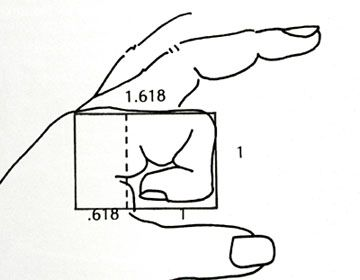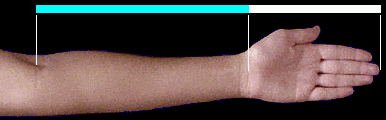The Golden Ratio and Human Body
Question:

The connection between mathematics and nature is sometimes stronger than people assume. Moreover, mathematics and psychology are also incredibly connected.

In this research, we are going to take a closer look at this unusual connection.

1. We will start with some measuring of our arm and index finger and find a certain ratio.
2. Then, we will learn the mathematical meaning of that ratio.
3. Finally, we will reflect on the connection between the new mathematical term and the way humans grasp the beauty.

Is there any connection between bones' ratio, human face, and mathematics? If so, what would it be?

1. Our index finger has three bones.
Each bone is followed by a smaller bone.
Now, divide the length of the long bone by the medium bone.
For example, 5 (the longest bone) / 3 (medium bone) =1.66
Insert your findings in the table attached to the map2. With a ruler, measure the length of your arm (from the elbow to the end of your longer finger) and your hand (palm and fingers).

Insert you findings in the table attatched to the mapThe ratio that you have discovered is called Phi number and it is found in nature and the human body.
The following mathematical explanation and link
will give you a deeper explanation about how we calculate Phi and how we find it in our body.
Think about the following sequence 1,1,2,3,5,8,13...
What is the next number? (Insert your findings in the table attached to the map)

Fibonacci discovered this mathematical sequence as part of biological research with rabbits.
The golden ratio is found in everyone's faces.
It seems that humans naturally (and unconsciously) defined beauty by intuitively assessing the golden ratio.
A face is considered more beautiful as ratios come closer to the Phi number.
In the next app, you can explore your beauty and origin.

Face's golden ratio on the computer  or  App which gives you golden ratio and origion
Remember, different types of beauty may exist but they all obey the same mathematical ratio- The Golden Ratio.

Results, Analysis and Discussion

 Points on map: